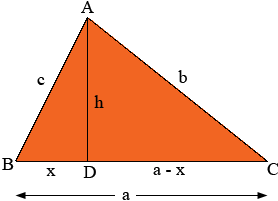SEARCH HOMEMath Central Quandaries & QueriesQuestion from GARIMA, a student: IF THE SIDES OF TRIANGLE ARE GIVEN . HOW WE WILL CALCULATE THE HEIGHT OF TRIANGLE.Garima,

If you want the height to calculate the area then you might calculate the area from Heron's formula directly. If you really want the height you can calculate the area using Heron's formula and then set

area = 1/2 × base × height

and solve for the height.

If you would rather not use Heron's formula you can use Pythagoras' theorem to set up two algebraic equations to solve for the height.BC is the base and h is the height. Triangle ABD is a right triangle and hence from Pythagoras' theorem

h2 = c2 - x2.

Triangle ADC is s right triangle so you can use Pythagoras' theorem again to write another expression for h2. Set the two expressions equal to each other and solve for x. Substitute into the expression above to find h.

PennyMath Central is supported by the University of Regina and The Pacific Institute for the Mathematical Sciences.